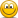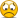# Now This Just Isn't Funny#### Frank Petrexa

##### Magistrate
I think that is not true. There is a numbering system which maps each rational number to an integer. Therefore there are countable infinite rational numbers.

Let's look at 1/0 . Well, what does x / y = a mean? Division is the inversion of multiplication, and x / y = a means x = a * y , and it is understood that this is a true and unequivocal statement.
Now with y = 0 you get x = 0 for every and each a, and this is neither reasonable nor unequivocal. So division by zero gives nonsense and is "forbidden"
Yes, Cantor came up with a way to map each rational number (a number with an integer in the numerator and another in the denominator, in lowest terms, where the numerator and denominator have no common divisors--1/137, 67/9000000, etc.) to an integer. So, the rationals are countable.
There is no such map from integers to real numbers.
The proof is as follows. Suppose there was. Then, one could arrange all the real numbers in order by the corresponding integer, just like Cantor did with the rationals. Just look at the interval between 0 and 1. If you can't count those, you can't count all the real numbers either. Take the decimal expansion of every number in there. They are in order based on the integer you've assigned to each number, so the first index is the order (i = 1, 2, etc.). The second is the decimal place (j = 1 is the 1/10 place, j=2 the 1/100 place, etc.)
So, each number in the list looks like 0.a(i,1)a(i,2)...a(i,i)a(i,i+1)... It is relatively easy to show that for EVERY real number, we can write a decimal expansion like this that is as close as we like to the value of the real number (I won't prove it here, and I'm sure everyone will be thankful for that, but I lifted it from Rudin, a mathematician born in Austria who was at the University of Wisconsin until his death in 2010).
Now, make a new number. The 1/10 place will be 6 if a(1,1) is 5, and 5 if it isn't. The 1/100 place will be 6 if a(2,2) is 5, and 5 if it isn't. The nth decimal place with be 6 if a(n,n) is 5, and 5 if it isn't. You get the idea.
This number is different than every number in the list. For any number in the list (for example the n = 37 millionth number), this number differs from it in a(n,n), the nth decimal place of the number in the nth slot in the list. So, it differs from EVERY number in the list in one of the decimal places. We built it that way. So, it can't be in the list, no matter how you made the list, and therefore you can't make a list of these real numbers that corresponds to the integers. There's always a number that isn't in there.

Yes, I guess this is way off topic and we need a "math" thread. There can't be enough crosses to crucify every real number.

Cantor came up with most of this stuff. (Goedel used this "diagonal" argument to show that any system of logic has at least one statement that cannot be proved or disproved using the system). People got really ticked off at Cantor (who suffered from depression). Kronecker in particular was scathing (he's the one who said "God made the integers, all the rest is the work of human hands" "Der Herrgot hat die reine Zahlen gemacht--alles anderes ist Menschenwerk"). So, "infinity" really isn't very well defined.

https://en.wikipedia.org/wiki/Controversy_over_Cantor's_theory

There are numbers that aren't rational, for example the square root of 2. Suppose we could write square root of 2 as a rational, say p/q. Both p and q are integers, and they can't have a common factor, so at least on of them is odd (not divisible by 2). Now, if we square p/q we get 2, because that's how we defined it. That means 2 = p**2/q**2, or 2(q**2) = p**2. The square of an odd number is not divisible by 2. So, if p was odd, the left side is divisible by 2 but the right side isn't. If p was even, the right side has a factor of 4, but the left side only has a factor of 2 (since q**2 isn't divisible by 2). This is impossible, so we can't write square root of 2 as p/q, no matter how hard we try. So, square root of 2 is not rational.

Last edited:

#### Loxuru

##### Graf von Kreuzigung
Yes, I guess this is way off topic and we need a "math" thread. There can't be enough crosses to crucify every real number.
And since every cross consists of two beams, we need twice as much beams as there are real numbers!#### phlebas

##### PRIMUS POENUS
Eva, that evil Temptress, that got man condemned to work, was navelless
I can't fault that logic.
Except to say that, as one created rather than born, she could have been created with a navel.

but here is the corrected versionI can't tell if this girl has a navel or not. Excuse me miss, could you . . . never mind, stay just right where you areLast edited:

#### Praefectus Praetorio

##### Senator
I can't fault that logic.
Except to say that, as one created rather than born, she could have been created with a navel.

but here is the corrected versionView attachment 707167
Owww. I love navels!